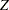The MULTTEST Procedure
 TEST Statement
TEST name ( variables </ options>) ;

The TEST statement is required unless the INPVALUES= option is specified. The TEST statement identifies statistical tests to be performed and the discrete and continuous variables to be tested. The following tests are permitted as name in the TEST statement.

CA

requests the Cochran-Armitage linear trend tests for group comparisons. The test variables should take the value 0 for a failure and 1 for a success. PERMUTATION= option can be used to request an exact permutation test; otherwise, a-score approximation is used. The CONTINUITY= option can be used to specify a continuity correction for the-score approximation.

FISHER

requests Fisher exact tests for comparing two treatment groups. The test variables should take the value 0 for a failure and 1 for a success.

FT

requests-score CA tests based upon the Freeman-Tukey double arcsine transformation of the frequencies. The test variables should take the value 0 for a failure and 1 for a success.

MEAN

requests the t test for the mean. The test variables can take on any numeric values.

PETO

requests the Peto mortality-prevalence test. The test variables should take the value 0 for a nonoccurrence, 1 for an incidental occurrence, and 2 for a fatal occurrence. The TIME= option should be used with the Peto test to specify an integer-valued variable giving the age at death. The CONTINUITY= option can be used to specify a continuity correction for the test.

If the value of a TEST variable is invalid, the observation is not used in the analysis. You can specify two tests only if one of them is MEAN. For example, the following statement is valid:

```test ca(d1-d2) mean(c1-c2);
```

But specifying both CA and FT, as shown in the following statement, is invalid:

```test ca(d1-d2) ft(d1-d2);
```

You can specify the following options in the TEST statement (some apply to only one test).

BINOMIAL

uses the binomial variance estimate for CA and Peto tests in their asymptotic normal approximations. The default is to use the hypergeometric variance.

CONTINUITY=number
C=number

specifies number as a particular continuity correction for the-score approximation in the CA and Peto tests. The default is 0.

LOWERTAILED
LOWER

is used to make all tests lower-tailed. All tests are two-tailed by default.

PERMUTATION=number
PERM=number

computes p-values for the CA and Peto tests by using exact permutation distributions when marginal success or failure totals within a stratum are number or less. You can specify number as a nonnegative integer. For totals greater than number (or when the PERMUTATION= option is omitted), PROC MULTTEST uses standard normal approximations with a continuity correction chosen to approximate the permutation distribution. PROC MULTTEST computes the appropriate convolution distributions when you use the STRATA statement along with the PERMUTATION= option.

DDFM= POOLED  |  SATTERTHWAITE

specifies whether the MEAN test uses a homogeneity assumption (DDFM=POOLED, the default) or deals with heterogeneous variances (DDFM=SATTERTHWAITE). See t Test for the Mean for more information.

TIME=variable

identifies the Peto test variable containing the age at death, which must be integer valued. If the TIME= option is omitted, all ages are assumed to equal 1.

UPPERTAILED
UPPER

is used to make all tests upper-tailed. All tests are two-tailed by default.Previous Page | Next Page | Top of Page